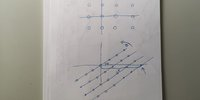# Find dimension of triangle

#### Dr.Peterson

##### Elite Member
Let's see if I can make sense of what you've said; the grammar of the last sentence is not at all clear.

You show a right triangle with legs $$\displaystyle 4s$$ and $$\displaystyle 5s$$ in the picture; I suppose the numbers 4 and 5 could be any integers, which you are calling #1 and #2. Let's call them $$\displaystyle a$$ and $$\displaystyle b$$ instead, so that in the picture $$\displaystyle a = 4$$ and $$\displaystyle b = 5$$; and I'll assume that they, too, are given, in addition to length $$\displaystyle s$$ and angle $$\displaystyle \theta$$.

The expression you wrote becomes $$\displaystyle \frac{as}{\sin(\theta)} - \frac{bs}{\cos(\theta)}$$. Can you explain your thinking? Is this supposed to equal x?

One problem I see is that $$\displaystyle a$$ and $$\displaystyle b$$ will determine $$\displaystyle \theta: \tan(\theta) = \frac{bs}{as} = \frac{b}{a}$$. So if $$\displaystyle \theta$$ is given, then you are assuming that its tangent is a rational number, which is not true in general. If you knew $$\displaystyle a$$ and $$\displaystyle b$$, rather than $$\displaystyle \theta$$, you could find $$\displaystyle \theta$$ from them.

Can you explain the requirements more fully?

#### Almeidammiguel

##### New member
Let's see if I can make sense of what you've said; the grammar of the last sentence is not at all clear.

You show a right triangle with legs $$\displaystyle 4s$$ and $$\displaystyle 5s$$ in the picture; I suppose the numbers 4 and 5 could be any integers, which you are calling #1 and #2. Let's call them $$\displaystyle a$$ and $$\displaystyle b$$ instead, so that in the picture $$\displaystyle a = 4$$ and $$\displaystyle b = 5$$; and I'll assume that they, too, are given, in addition to length $$\displaystyle s$$ and angle $$\displaystyle \theta$$.

The expression you wrote becomes $$\displaystyle \frac{as}{\sin(\theta)} - \frac{bs}{\cos(\theta)}$$. Can you explain your thinking? Is this supposed to equal x?

One problem I see is that $$\displaystyle a$$ and $$\displaystyle b$$ will determine $$\displaystyle \theta: \tan(\theta) = \frac{bs}{as} = \frac{b}{a}$$. So if $$\displaystyle \theta$$ is given, then you are assuming that its tangent is a rational number, which is not true in general. If you knew $$\displaystyle a$$ and $$\displaystyle b$$, rather than $$\displaystyle \theta$$, you could find $$\displaystyle \theta$$ from them.

Can you explain the requirements more fully?
The expression i wrote i was just thinking in how to start the problem! Dont mind it it might not be anything usefull!

The requirement is: if i have a grid of circles and rotate this grid being the rotation center one circle, somewhere there will be a circle that is completly aligned in the axis direction with the circle that serves as rotation centre - Image in attachment.Thats why i discrimined the tan $$\displaystyle \theta=\frac{a}{b}$$ because the grid cannot change!

#### HallsofIvy

##### Elite Member
It looks to me like this is over determined. You have legs of length 4s and 5s and angle $$\displaystyle \theta$$ opposite the 5s side. From that $$\displaystyle tan(\theta)= \frac{5s}{4s}= \frac{5}{4}$$ so that $$\displaystyle \theta= 51.3$$ degrees yet you say that $$\displaystyle \theta$$ is "given"!

#### Dr.Peterson

##### Elite Member
It appears that you are missing the fact that your s is irrelevant, since the angle depends only on the ratio so that s cancels out. There is going to be "somewhere there will be a circle that is completely aligned" if the tangent of your angle happens to be a rational number. That is true only for very special angles. Going out further to look doesn't help, because of the cancellation.